# How to Sum Across Multiple Sheets in Microsoft Excel

In this article, we will learn how to get the sum or add cells across multiple sheets in Microsoft Excel.

Sometimes we need to access different values from different worksheets of the same excel book. Here we are accessing it to add multiple cells in Excel 2016.

In this article, we will learn how to sum the values located on different sheets in excel 2016. We will use the SUM function to add numbers.
SUM function adds up the values.
SUM = number 1 + number 2 + …
Syntax:

 =SUM(number 1, number 2, ..)

Let’s understand how to add cells in excel 2016 with the example explained here.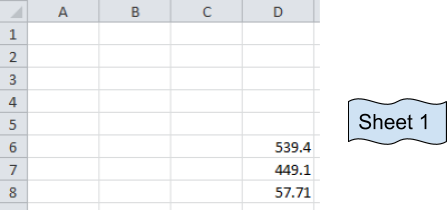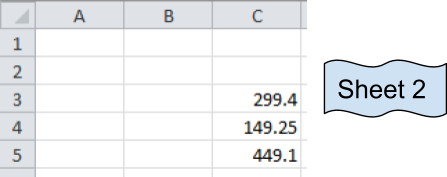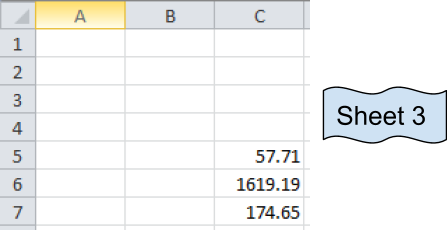These are numbers from three different sheets and desired output sum will be in Sheet 1.

Now we use the SUM function

Formula:

 =SUM(D6:D8, Sheet2!C3:C5,Sheet3!C5:C7)

Explanation:
The resulting output is in Sheet 1.
D6:D8 adds the values of Sheet 1  D6+D7+D8
C3:C5 adds the values of Sheet 2  C3+C4+C5
C5:C7 adds the values of Sheet 3   C5+C6+C7
It's basically the addition of values in cells
D6+D7+D8 + C3+C4+C5 + C5+C6+C7

You can select the cells separated by commas to add the numbers.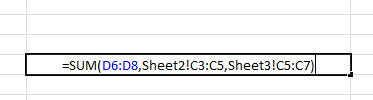Your formula will look like the above image.

Press Enter and your desired sum will be here in Sheet 1.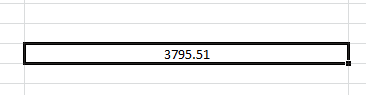As we can see Sum function returns the sum. You can use Autosum option in Home tab in Editing.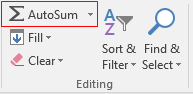Click arrow key for more options like shown below.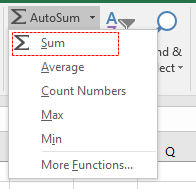Then select the cells to add up values in Excel. You can sum across the rows and columns using the SUM function.

Hope you got SUM function adding cells in excel. The same function can be performed in Excel 2016, 2013 and 2010. Let us know how you like this article. You will find more content on functions and formulas here. Please state your query down in the comment box. We will help you.

If you liked our blogs, share it with your friends on Facebook. And also you can follow us on Twitter and Facebook. Hope you got SUM function adding cells in excel. The same function can be performed in Excel 2016, 2013 and 2010. Let us know how you like this article. You will find more content on functions and formulas here. Please state your query down in the comment box. We will help you.
We would love to hear from you, do let us know how we can improve, complement or innovate our work and make it better for you. Write us at info@exceltip.com

Popular Articles:

50 Excel Shortcut to Increase Your Productivity

How to use the VLOOKUP Function in Excel

How to use the COUNTIF function in Excel

How to use the SUMIF Function in Excel

1.krishna Moorthy

I have an excel workbook with the each sheet having the total amount in a different cell like D3, D12, D18 etc. i have 30 sheets like that. Now i need a generic formula to add those column without mentioning the rows and get the sum in the next sheet.

•Hi krishna,
You must have some way to identify which cells contain the total in each sheet. The best way to do this is to have them in the same cell of each sheet. However if it needs to be in different cells, you can use name_ranges. Name each total cell with their sheet name. Like Sheet1_total, Sheet2_total, Sheet3_total, and so on. In the grand sum formula, use formula `=SUM(Sheet1_total,Sheet2_total, Sheet3_total....SheetN_total)` This will solve the issue and it will be dynamic too. You can read about named ranges here https://www.exceltip.com/excel-range-name/all-about-excel-named-ranges-excel-range-name.html

2.Farmula of Ashish worked fine

3.Thank you! worked and very useful.

Can you please tell which formula works when there are hidden cells.

4.thank you

5.I love sheets

6.I have a small store of materials. and having about 300 employees, who use to take material on daily base. i have made different sheets for each employee. there are about 30 materials. and the materials are repeated for each of employee several times.

i need a general format in excel, that can calculate by looking exact material name and just sum it up for all the 300 employees. and show me the exact issued amount of each material individual.

7.I have this formula ='1-1'!B1 that links the total on sheet 1-1 to the main sheet. How do I copy the formula so it link like the totals on sheets 1-2, 1-3 1-4 all the way to 12-31? (For the whole year)? All the to totals are on Cell B1on each sheet. The only thing that changes is the sheet number: 1-1, 1-2, 1-3 etc..

8.mohit agarwal

any body tell me
how to hide formula
bt i wanna formula will work depend afetr hide

•Ramandeep Singh

Hi Mohit Agarwal,

For hiding the formula, select the range / cells containing the formulas that need protection. Right click and choose format cells, then go to the Protection Tab. In Protection Tab, select LOCKED and HIDDEN check box and click on OK (Locked check box restricts editing of cell). Then, press the combination of Alt + T + P + P keys, a Protect Sheet option window will appear. Apply password, if required and click on OK.

9.I would like to use the =SUM(Sheet1:Sheet15!) type sum for a range of worksheets, but not the same cells on each worksheet. I see above this can obviously be done by typing them in separately, however, I would like to use the range of worksheets. This is because I often add more tabs in between Sheet1 and Sheet15 and I want all my SUM formulas to include these new tabs without having to change every formula. This is a very large excel file with a LOT of formulas that would need to be changed every time. Any help?

10.Thanks It working very well

•Thanks Asif for the appreciation. 🙂

11.It works, thanks! Another tip if I may. If you name your sheet as numbers (1,2,3,...) the formula should be like this: =SUM('(SHEET1):(SHEET2)'!CELL NUMBER)
example:
=SUM('(1):(27)'!M39)

•Thank you!

12.How do you copy from multiple sheets if it a formula you want to total? (Instead of just a number in the cell)

13.Thanks, this was very and I mean very helpful

14.What if the total in the separate work sheets are not in the same cell on each worksheet?

For example some are in C38 and some are in C43 and so on..

How do I get the totals from each worksheet?

•Hi Jannae,

In case you are working with 2 sheets i.e. Sheet1 & Sheet2.
Case#1: Cell C38 & C43 in both sheets has values; to get total of these values we can use formula =SUM(Sheet1!C38,Sheet1!C43,Sheet2!C38,Sheet2!C43)

Case#2: Cell C38 has some value in both sheets while cell C43 has value in Sheet1 only in that scenario the formula =SUM(Sheet1!C38,Sheet1!C43,Sheet2!C38)

Regards,
Ashish

•WHAT IF YOU WANT TO DRAG THE FUNCTION BECAUSE WHEN I DRAG IT USES ONLY THE CELL THAT I USED IN THE PREVIOUS WORKBOOK

•Thank you so much Ashish for the explanation. it is well noted.

15.i have 5 sales man whose selling data is put in one mixed column per day randomly ( not neccesary their sale amount is one by one in a column ).
now i want to auto update their personal selling automatically in another excell sheet while i enter any salesman selling amount in first page with his name.

please need help through e-mail as soon as possible.

•Hi,

Thanks for contacting us!

Please post your query @ www.excelforum.com. There you can attach Excel file so it would be easier to understand the query. And if you can share the excel file then we will resolve the query here only.

Thanks
Excel Tip & Excel forum team

16.This was very helpful! Worked like it was explained. Thank you!

17.my question is in my office every day some data enter in day one and anther day second sheet ext. totally 30 day 30 sheet per month my question i want report totally particular sheet in display

18.How do you add numbers in cells in different positions on different sheets? I thought I was doing so, but end up with some cells being automatically selected on subsequent sheets (often empty ones, but not always).

•How do you add numbers in cells in different positions on different sheets? I thought I was doing so, but end up with some cells being automatically selected on subsequent sheets (often empty ones, but not always).

19.Thanks. It works

20.I get a circular error when I do this. Please help

•I had this happen too until I took out any spaces from the tab name (Team 1 needed to be Team1). Then it worked as advertised.

•I did this however it sum the first tab number and no other tab amounts.

••